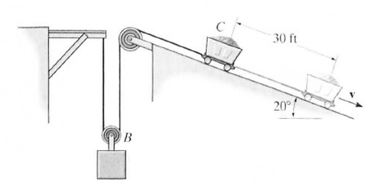# The car C and its contents have a weight of 600 lb, whereas block B has a weight of 270 lb. The...

## Question:

The car C and its contents have a weight of 600 lb, whereas block B has a weight of 270 lb. The car is released from rest. Determine the car's speed when it travels 30 ft down the 20{eq}^\circ {/eq} incline. Suggestion: To measure the gravitational potential energy, establish separate datums at the initial elevations of B and C.## Principle of Conservation of Energy:

According to principle of conservation of energy, the energy can neither be created nor be destroyed and the energy can be changed from one for to the other. Mathematically this can be written as,

{eq}T_1+V_1= T_2+V_2 {/eq}.

{eq}\textbf {Given:} {/eq}

• Weight of the car, {eq}W_c=600\ \text {lb} {/eq}
• Weight of the block, {eq}W_b=270\ \text {lb} {/eq}
• Distance travelled, {eq}s_c=30\ \text {ft} {/eq}

Since the length (L) of the cable remains same even after the motion of the car, we can write the length of the cable as

{eq}L=2s_b+s_c\\ 2\delta s_b+\delta s_c=0\\ 2\delta s_b=-\delta s_c\\ 2\delta s_b=-30\\ \delta s_b=-15\ \text {ft} {/eq}

Apply the work-energy principle to get the velocity of the car C.

{eq}T_1+V_1= T_2+V_2\\ \dfrac {1}{2}m_bv_{b1}^2+\dfrac {1}{2}m_cv_{c1}^2+V_{b1}+V_{c1}=\dfrac {1}{2}m_bv_{b2}^2+\dfrac {1}{2}m_cv_{c2}^2+V_{b2}+V_{c2}\\ 0+0+0+0=\dfrac {1}{2}\left (\dfrac {270}{32.2}\right )v_{b2}^2+\dfrac {1}{2}\left (\dfrac {600}{32.2}\right )v_{c2}^2+(270\times 15)+600(-30\times\sin 20^\circ)\\ 0=\dfrac {1}{2}\left (\dfrac {270}{32.2}\right )\left (\dfrac {-v_c}{2}\right)^2+\dfrac {1}{2}\left (\dfrac {600}{32.2}\right )v_{c2}^2+(270\times 15)+600(-30\times\sin 20^\circ)\\ 0=10.36v_c^2-3434.36\\ \bf \color {navy} {v_c=18.21\ \text {ft/s}} {/eq}